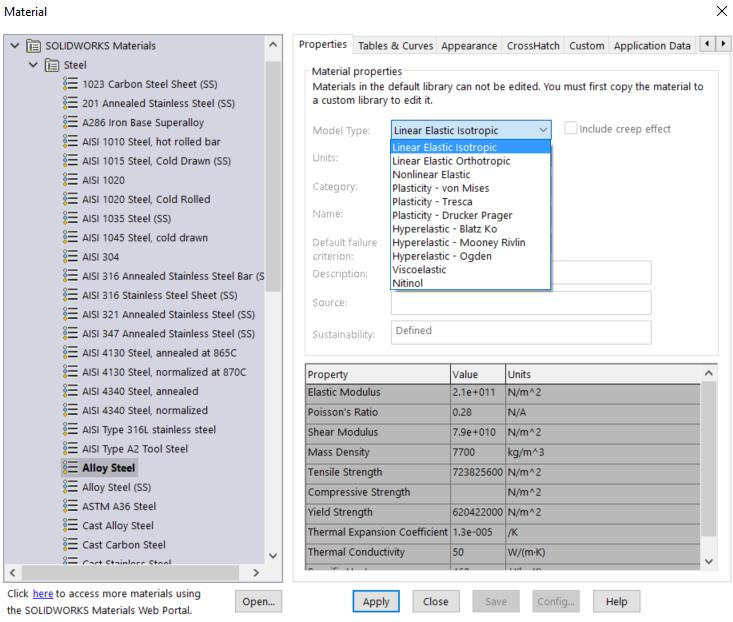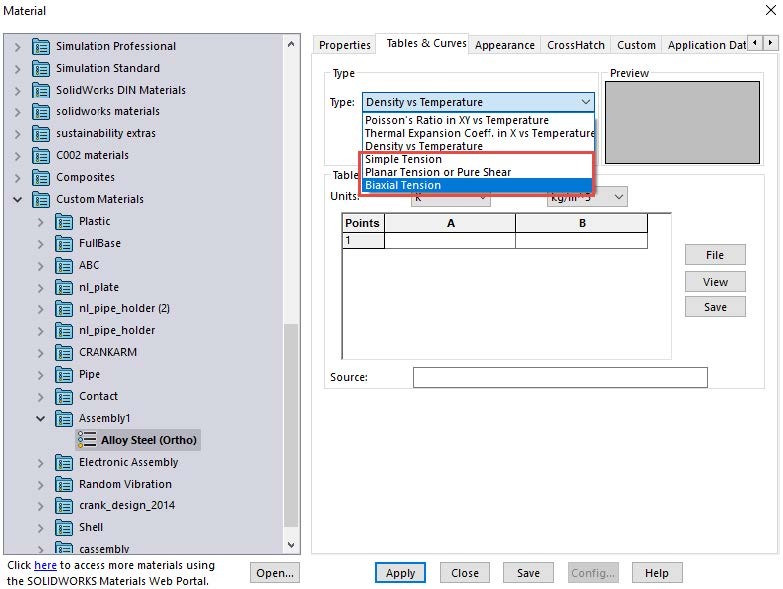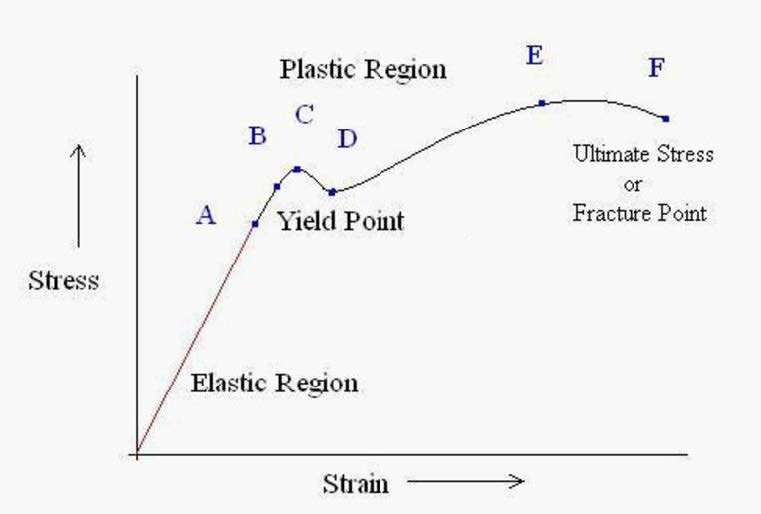# SOLIDWORKS Simulation Premium Material Model Types Defined

Article by Taran Packer on Aug 09, 2015

SOLIDWORKS Simulation Premium has 11 material model types that will influence the results of a Linear or Nonlinear Analysis. These models types are located in the Properties tab in the material properties (See Figure 1) and will be defined individually in this article. These definitions are not comprehensive. They are only intended to give SOLIDWORKS Simulation Premium users a general understanding of how each material model works in the solver.Figure 1: List of the Material Model Types in SOLIDWORKS Simulation Premium

The top three material models listed (See Figure 1) are elastic material models. This means that they are only accurate in the elastic zone of the stress-strain curve (see figure 3) of the material that is being applied to the model in the study. As long as the material being put under load has a max stress that is still in the elastic region it will return to the pre-load state once the load has been released.

## SOLIDWORKS Simulation Premium Material Model Types

• Linear Elastic Isotropic should be applied to materials that are materially the same in the X, Y, and Z direction, and is defined by two material constants (Elastic’s modulus and Poisson’s ratio). This means that if the user were to apply a force in the X, Y, or Z direction, individually all three axes would show the same results equally.
• Linear Elastic Orthotropic exhibits different material properties and behaviors in all three Cartesian Coordinates axes and has nine different material constants.
• Nonlinear Elastic – While this material is elastic it does exhibit the property of returning to its preloaded state post load. It does not have a linear region. It exhibits nonlinearity from the very beginning of the load being applied to the object.

### Hyper-Elastic Material Models

Hyper-Elastic material models have the capacity to take large amounts of strain while exhibiting relatively minor stress. These types of materials can deform significantly under small loads. Rubber is a really good example of this type of material model. Materials that fit this model can exhibit highly complex behaviors that can be simulated in the program to a high degree of accuracy if the proper elastic constants are input into the material CommandManager. The user can input 2, 5, or 6 constants. With each correct elastic constant being placed into the program, a higher level of accuracy can be noticed in the solution.

These elastic constants are found through experimentation and are generally provided by the manufacturer. If they are not provided, the user has the option to input up to three stress-stretch curves into the Tables & Curves tab. These curves can be accessed by clicking on the Type drop-down menu (See Figure 2) and are entered exactly like a stress-strain curve. Once these curves have been provided the program will automatically calculate the proper elastic constants for the material from the curves. Only one of the three curves is required but the more curves that are provided by the user the better the elastic constants can be calculated, and the greater the accuracy of the material model.Figure 2: Three stress-stretch curves available in hyper-elastic material models.

• Hyper-Elastic Blatz Ko is used to simulate compressible hyperelastic materials such as foam. It only requires one elastic constant, and the elastic modulus of the material to calculate an accurate result. The Poisson’s ratio is assumed to be 0.25 by default for this material model.
• Hyper-Elastic Mooney - Rivlin is one of the most popular hyper-elastic material models because of the high amount of experimentation that has been done to these types of materials. This material model has a greater number of available constants overall provided by the manufacturer of the material. This material has the option of inputting all six possible elastic constants but all six are not required, just suggested for greater accuracy.
• Hyper-Elastic Ogden uses an elastic strain energy density function that is considered to be the most accurate at simulating very large deformation in rubber-like materials.

### Elasto-Plastic Material Models

Elasto-Plastic models become necessary after the material being simulated reaches the yield point and enters the plastic range of the materials stress-strain curve (See Figure 3). Once in the plastic region, the object being deformed will not return to its original configuration after the load on the object has been removed.

The deformation at this point will be permanent. If the load is then placed back on the object it will not follow the original loading path but the previous unloading path back up into the plastic region. The yield point will have also moved to a higher stress location than it was originally, this is what is known as material hardening. As the object is loaded and unloaded into the plastic region the load path changes so that the same stress results give different strain results.

• Plasticity – von Mises the material starts to yield when the Von Mises stress at a single point reaches a specific experimentally known constant scalar value called the yield strength of the material. This material model is widely used and is especially accurate when being used with a ductile material like metal.
• Plasticity – Tresca the material starts to yield when the maximum shear stress material equals the specific experimentally known maximum shear stress at a single point in the model for that material. The Tresca model is generally more conservative than the von Mises model and Tresca does not support large strain plasticity formulation.
• Plasticity – Drucker-Prager is good at simulating the behavior of granular soil materials like sand or gravel. Only two parameters are absolutely necessary. The angle of internal friction, and the material cohesion strength. Like the Tresca model this material model also does not support large strain plasticity formulation.Figure 3: Typical Stress-Strain Curve

### Super-Elastic Material Models

• Nitinol is the only Super-Elastic Material Model in SOLIDWORKS. If the end-user is trying to find the Super Elastic category in the material model types they will not find it. This category is under the word Nitinol in SOLIDWORKS Nonlinear Simulation.

### Viscoelastic

Viscoelastic is the only material model type that is time-sensitive. All of the other material models used in SOLIDWORKS Simulation Premium will show the exact same loading stress-strain path no matter how quickly or slowly the load is applied. Viscoelastic materials are different they are strain-rate dependent meaning a load of equal magnitude applied to this material model at a different rate will produce a different stress-strain curve even if the max magnitude remains the same.

## Related SOLIDWORKS Simulation Articles

SOLIDWORKS Simulation: Missing material data? No problem!

SOLIDWORKS Simulation Nonlinear Material Models Stress-Strain Curves Explained

SOLIDWORKS Simulation Composite Analysis

SOLIDWORKS Simulation Gauss Points, Nodal, and Element Stress

Piecewise Nonuniform Pressure in SOLIDWORKS Simulation